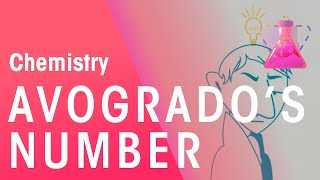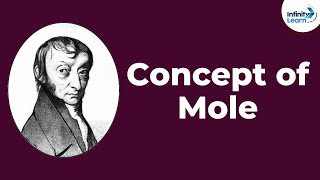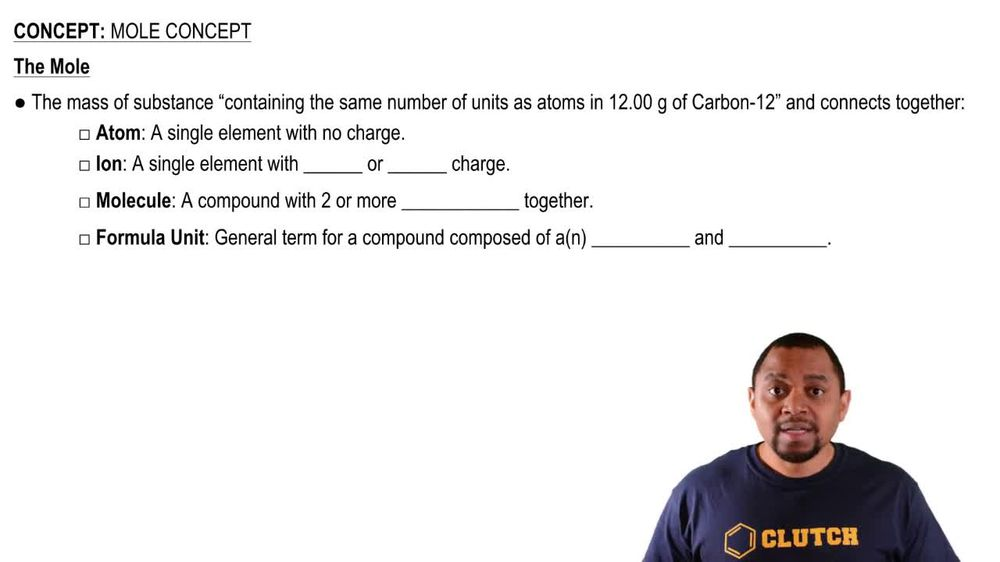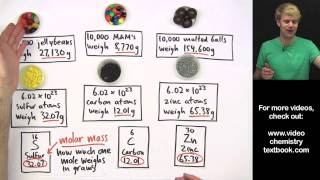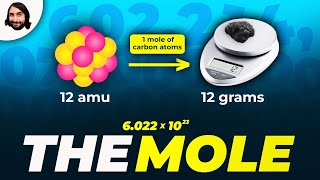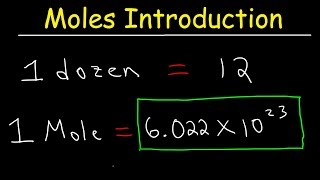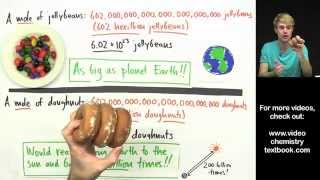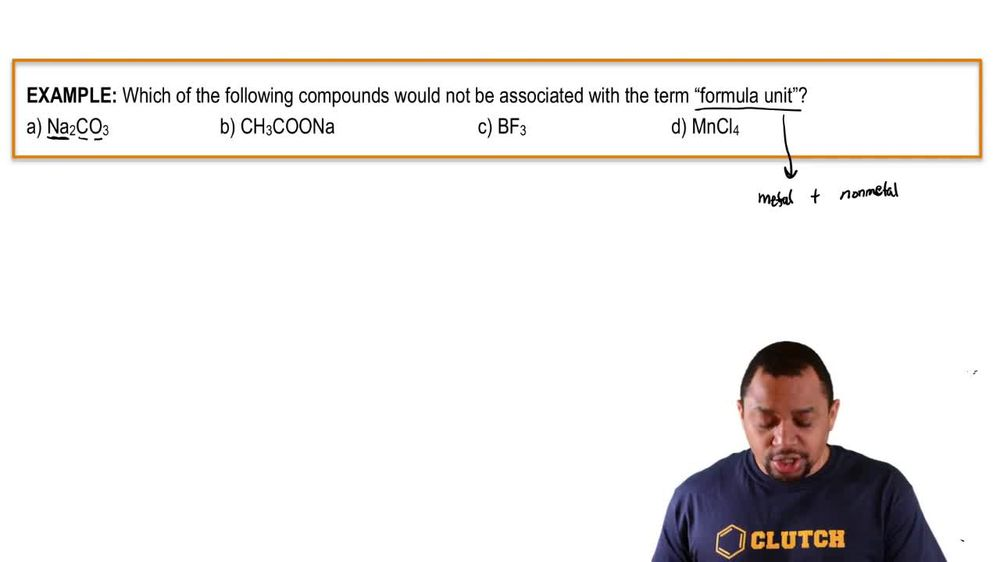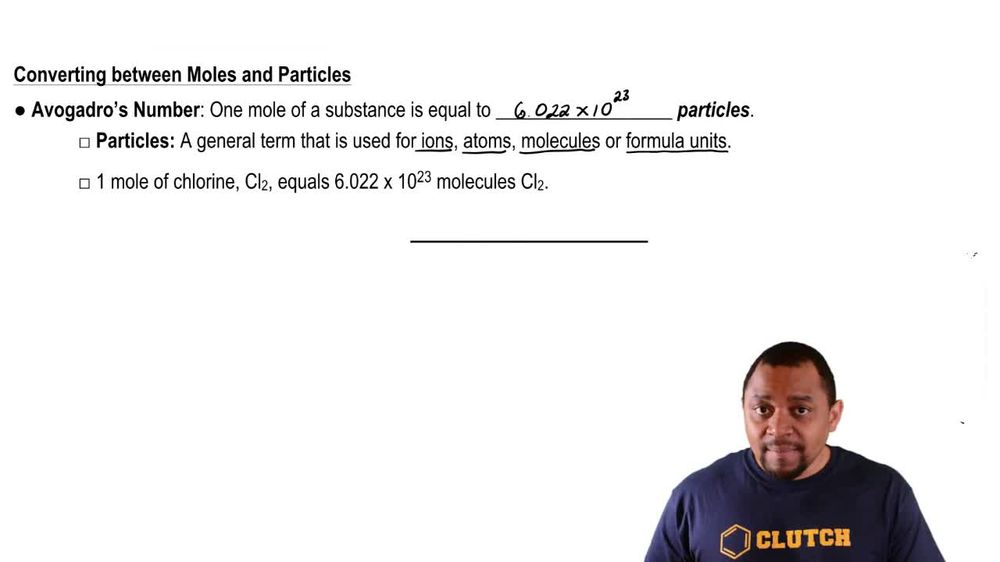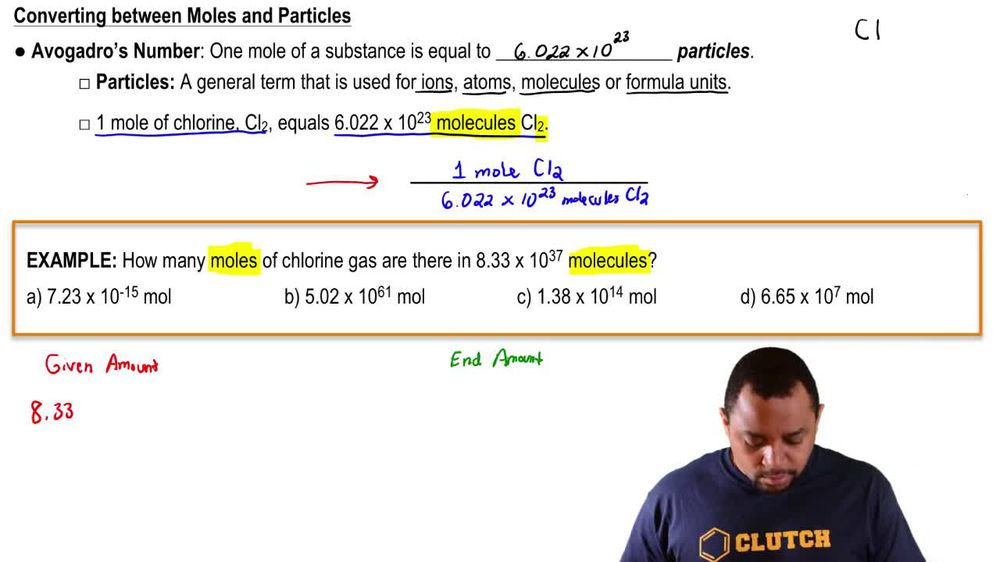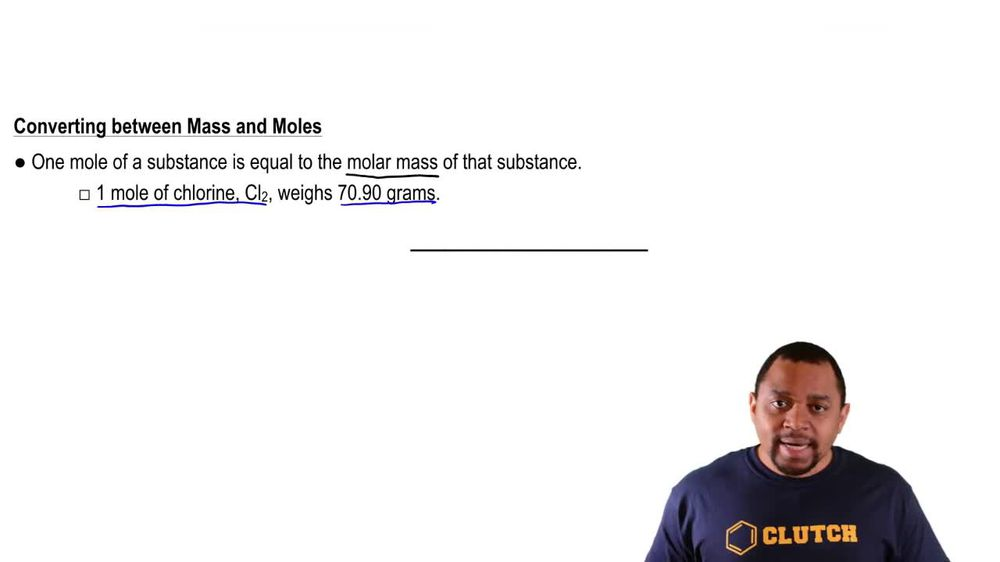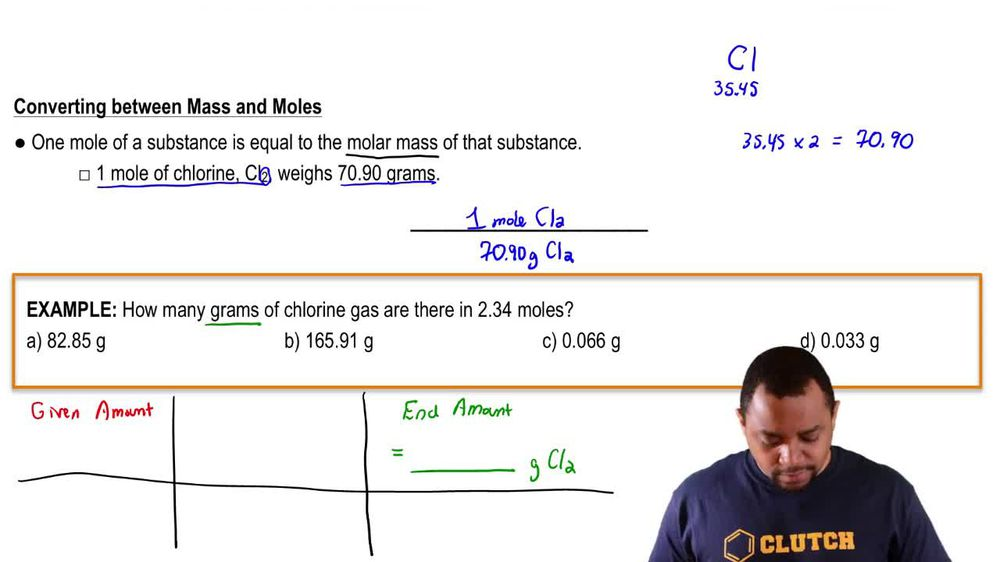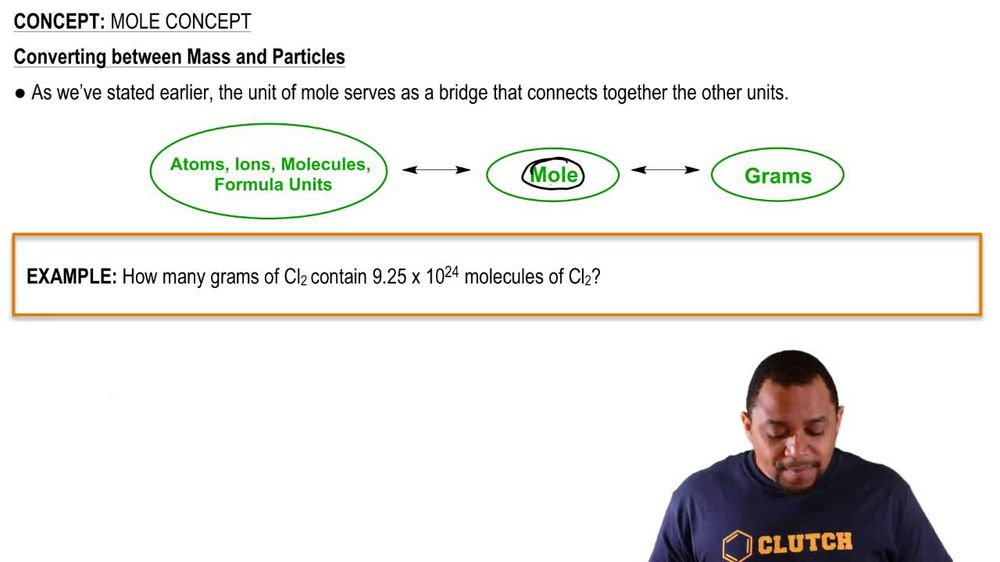Start typing, then use the up and down arrows to select an option from the list.
1. 2. Atoms & Elements2. Mole Concept
Problem

# The molar mass of HCl is 36.5 g/mol, and the average mass per HCl molecule is 36.5 u. Use the fact that 1 u = 1.6605 * 102 24g to calculate Avogadro’s number.

Relevant Solution2m
Play a video:
Hello everyone today. We have the following question derive an accurate value. Up to four significant figures. The average joe's number. If the average mass of a molecule of oxygen is units and the molar mass of oxygen is 32 g per mole. Recall that one unit is equal to 1.661 times 10 to the negative 24 grams. So it's important to recall what avocados number is. So avocados number is simply the number of molecules or atoms or ions that are in one mall of anything. And so the mass of an oxygen molecule I stated in the question is going to be 32 units per molecule. And what we're gonna do with this value, you're going to multiply that by the one U. That's equal to that. 1.6 61 times 10 to the -24g. You interview, we're going to cancel out and then we're going to Calculate that. And we will be left with 5.3136 times 10 to the negative 23rd g per molecule. But we aren't done just yet. Our last and final step we'll be finding avocados number with that mass. And so that's going to be equal to 32 g per one mole which is the molar mass of one oxygen molecule. And oxygen gas molecule. We're going to multiply that by the conversion factor that one molecule Is equal to 5.3136 times 10 to the negative 23rd g arguments of grand will cancel out And it will be left with 6.02, 2 times 10 to the 23rd molecules Permal. And this will be our final answer. Overall, I hope this helped, and until next time.